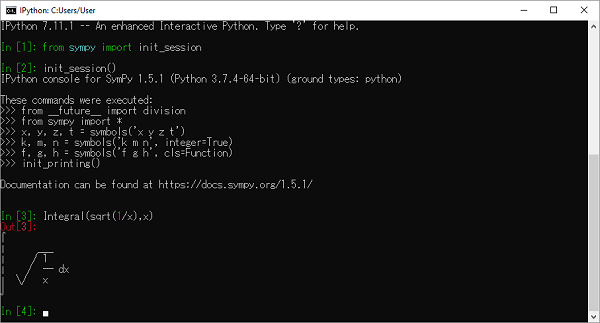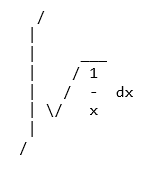# SymPy - Printing

There are several printers available in SymPy. Following is a partial list −

• str
• srepr
• ASCII pretty printer
• Unicode pretty printer
• LaTeX
• MathML
• Dot

SymPy objects can also be sent as output to code of various languages, such as C, Fortran, Javascript, Theano, and Python.

SymPy uses Unicode characters to render output in form of pretty print. If you are using Python console for executing SymPy session, the best pretty printing environment is activated by calling init_session() function.

>>> from sympy import init_session
>>> init_session()


IPython console for SymPy 1.5.1 (Python 3.7.4-64-bit) (ground types: python).

These commands were executed −

>>> from __future__ import division
>>> from sympy import *
>>> x, y, z, t = symbols('x y z t')
>>> k, m, n = symbols('k m n', integer=True)
>>> f, g, h = symbols('f g h', cls=Function)
>>> init_printing()


Documentation can be found at https://docs.sympy.org/1.5.1/.

>>> Integral(sqrt(1/x),x)


$\int \sqrt\frac{1}{x} dx$

If LATEX is not installed, but Matplotlib is installed, it will use the Matplotlib rendering engine. If Matplotlib is not installed, it uses the Unicode pretty printer. However, Jupyter notebook uses MathJax to render LATEX.

In a terminal that does not support Unicode, ASCII pretty printer is used.To use ASCII printer use pprint() function with use_unicode property set to False

>>> pprint(Integral(sqrt(1/x),x),use_unicode=False)The Unicode pretty printer is also accessed from pprint() and pretty(). If the terminal supports Unicode, it is used automatically. If pprint() is not able to detect that the terminal supports unicode, you can pass use_unicode=True to force it to use Unicode.

To get the LATEX form of an expression, use latex() function.

>>> print(latex(Integral(sqrt(1/x),x)))


\int \sqrt{\frac{1}{x}}\, dx

You can also use mathml printer. for that purpose, import print_mathml function. A string version is obtained by mathml() function.

>>> from sympy.printing.mathml import print_mathml
>>> print_mathml(Integral(sqrt(1/x),x))


<apply>

<int/>

<bvar>

<ci>x</ci>

</bvar>

<apply>

<root/>

<apply>

<power/>

<ci>x</ci>

<cn>-1</cn>

</apply>

</apply>

</apply>

>>>mathml(Integral(sqrt(1/x),x))


'<apply><int/><bvar><ci>x</ci></bvar><apply><root/><apply><power/><ci>x</ci><cn>-1</cn></apply></apply></apply>'